# Very useful Math Apps on Android for everyone

With everything going on, doing mental calculations and solving complex equations or making graphs could be a tedious task. In such scenarios, a great number of applications on Google Playstore  will surely help you in remembering the correct formulas and in turn allow you to calculate any complex function or equation on the go. Have a field job which involves doing various calculations on PC? Just install these enlisted apps on your Android device and you can do every calculation on the go.

### 1. Mathematics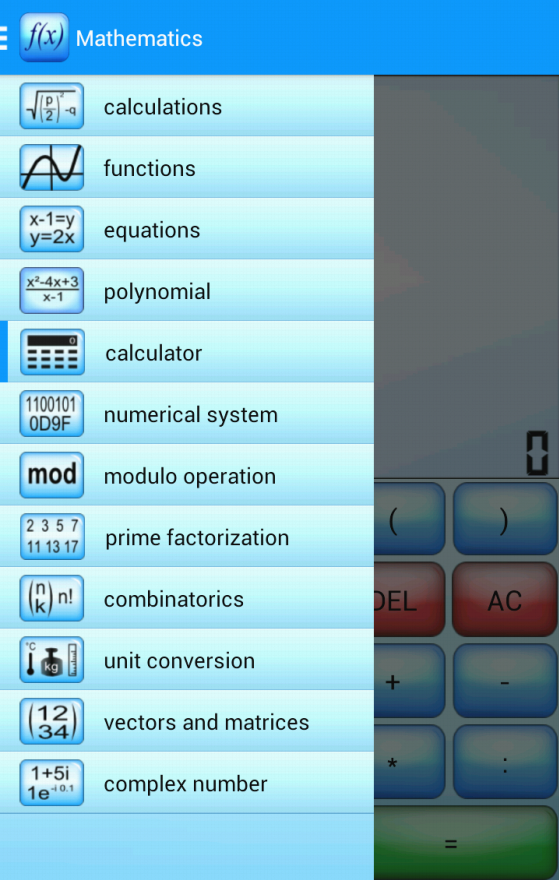Termed as powerful mathematics software, this app allows you to calculate any formula you want and show them in a 2d or 3d plot. You can derive or integrate any desired function with in seconds plus calculate the zero points of your function and show them in the function plot as well.

Android Version File Size Price Google Playstore
2.2 2.5(MB) Free Mathematics

### 2. WolframAlpha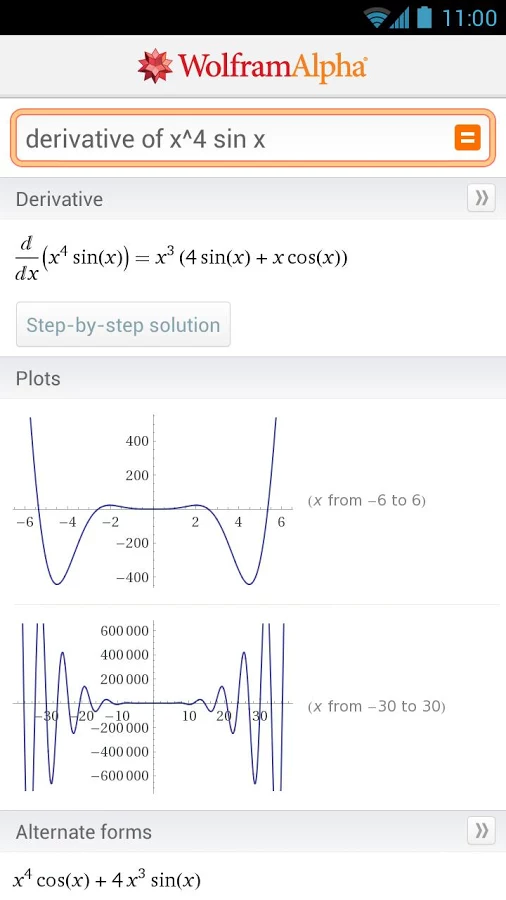Wolfram|Alpha has rapidly become the world's definitive source for instant expert knowledge and computation. This application mainly uses its vast collection of algorithms and data to calculate the answers and then generate reports for the user.

Android Version File Size Price Google Playstore
2.1 2.2(MB) \$2.99 WolframAlpha

### 3. Matrix Operations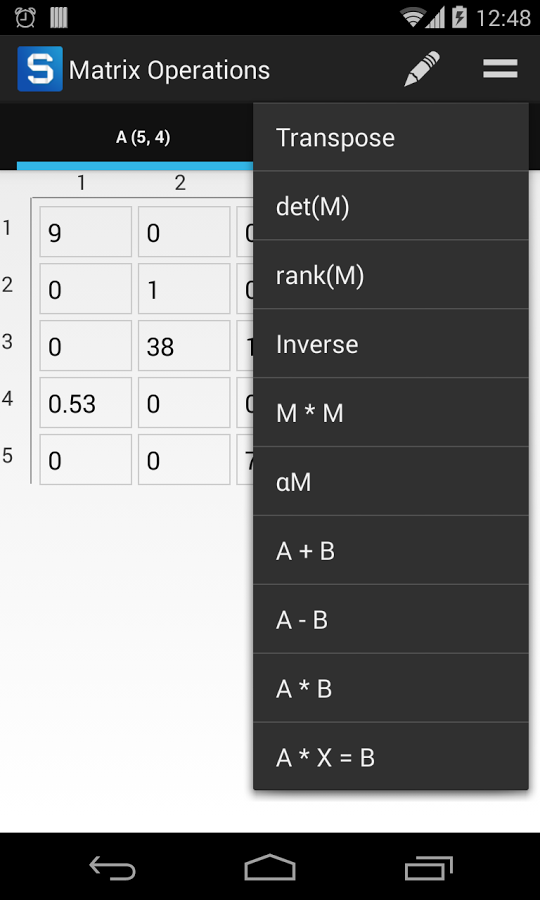As the name suggests, Matrix Operations is a valuable application if you are performing simple operations and calculations with matrices. You can easily calculate determinant,  sum and diff of matrices and solve linear equations (with Gauss algorithm) A*X=B.

Android Version File Size Price Google Playstore
2.3 1.6(MB) Free Matrix Operations

### 4. Math Ref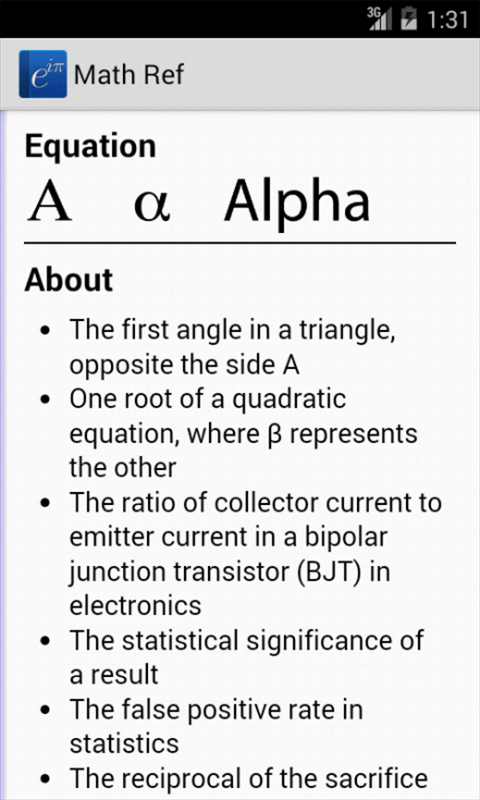A very comprehensive app which allows its user to browse over 1,400 formulas, figures, and examples. The numerous examples prove quite helpful in understanding the formulas. Various tools such as unit converter, quadratic solver, and triangle solver provide further advantage to the user.

Android Version File Size Price Google Playstore
4.0 19(MB) \$0.99 Math Ref

### 5. MyScript Calculator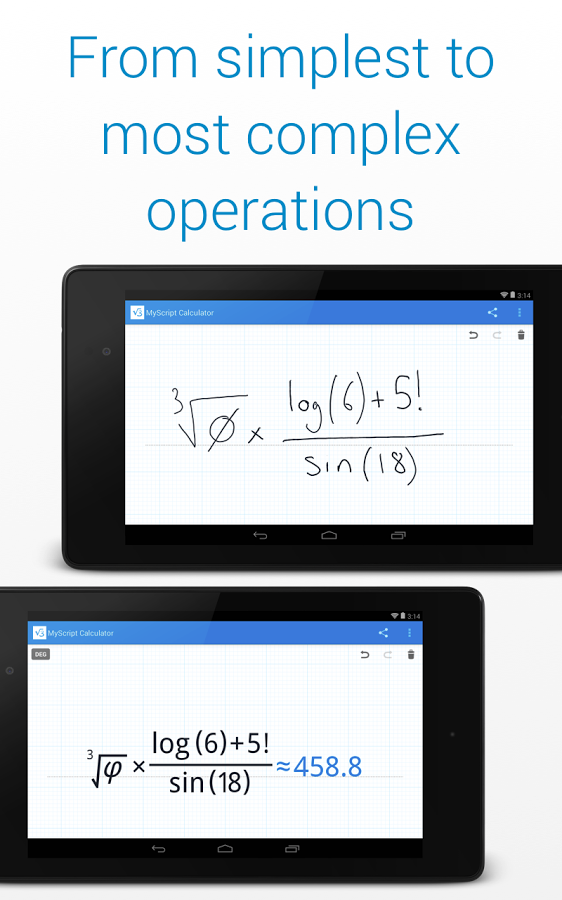MyScript Calculator allows you to solve mathematical equations by hand without actually having to crunch the numbers yourself. The application provides the same experience as writing on paper but with a technological touch. Just write the mathematical expression on the screen then let MyScript Calculator perform its magic.

Android Version File Size Price Google Playstore
Varies with devices Varies with devices Free MyScript Calculator

### 6. Graphing Calculator by MathlabAn amazing app since it not only provides you with the answer but rather breaks it down for your understanding. Graphing Calculator has algebra integration in it as well plus it provides multiple functions on a graph, polar graphs, graphing of implicit functions, values and slopes, roots, extremes, intersections.

Android Version File Size Price Google Playstore
2.1 1.9(MB) Free Graphing Calculator by Mathlab

### 7. Graphing Calculator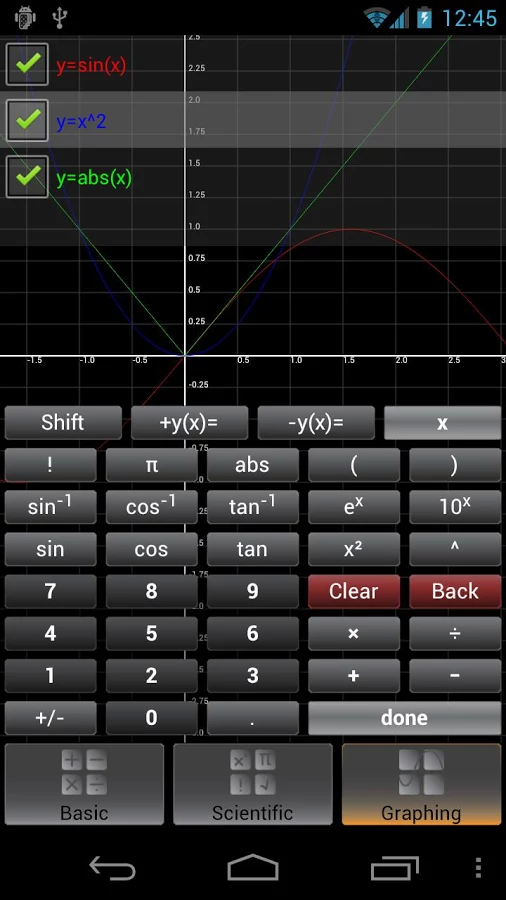A simple graphing calculator application that allows for easy scientific calculations. You can take screenshots, copy and paste using Clipboard and you can also press Shift on the top left corner in case you don't find any operation key.

Android Version File Size Price Google Playstore
2.1 220(KB) Free Graphing Calculator

### 8. Equation Solver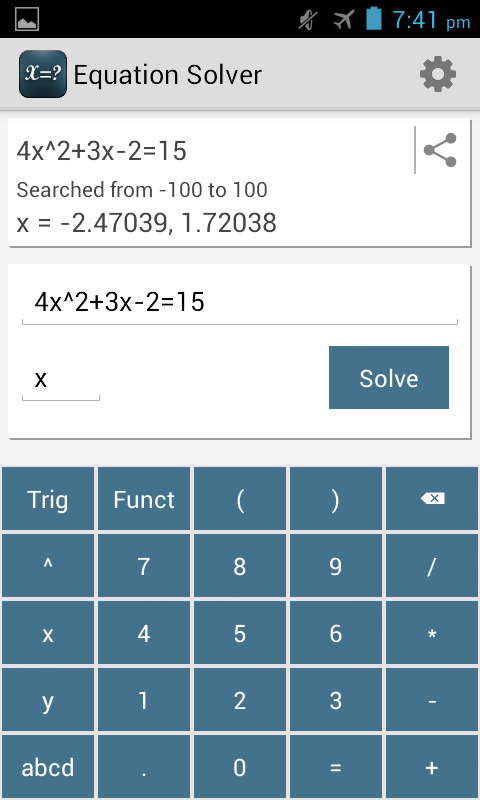Equation Solver is an application that is not limited to a formula rather solves every kind of equation. This app uses the brute force method instead of using the coded formulas. An ideal application for quickly solving any equation in a relatively small amount of time.

Android Version File Size Price Google Playstore
2.1 1.7(MB) Free Equation Solver

### 9. Linear Equation System Solver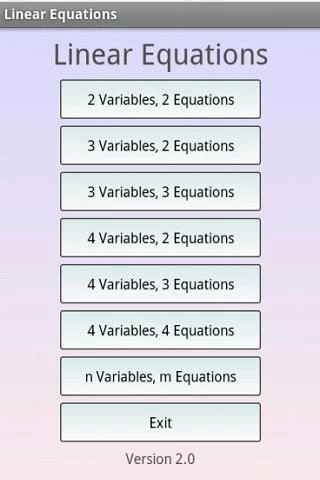Have difficulty in solving linear equations? Well this is the right kind of app for you. It provides with you with the mathematical tools for solving linear equations which have a major role in the fields of Engineering, Physics, Chemistry and Computer Science.

Android Version File Size Price Google Playstore
1.6 814(KB) Free Linear Equation System Solver

### 10. Math Formulas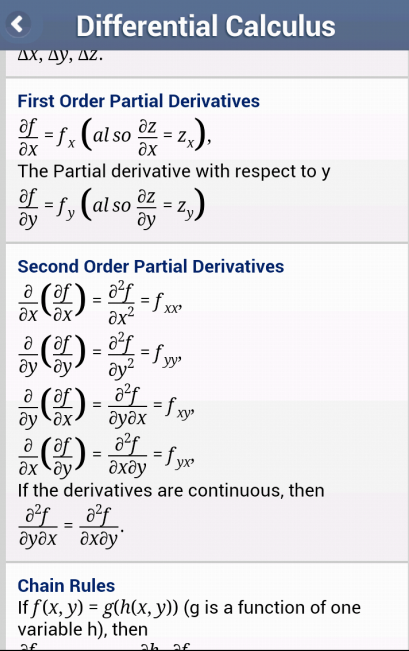Continuous updation of details and frequently added new topics are a major attraction of this app. Math Formulas covers all the basic topics related to maths and allows for horizontal swipe on individual formulas if content is not fully displayed. This application is one of a kind where mathematical formulas are aggregated in a useful way.

Android Version File Size Price Google Playstore
2.2 3.0(MB) Free Math Formulas

### BONUS. Math Workout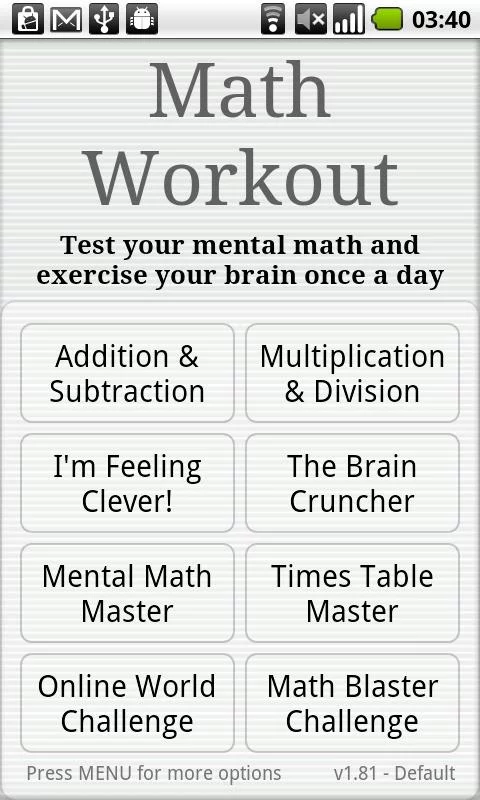Exercising for physical fitness is one thing but exercising for mental fitness is another. Math Workout is an app designed to keep the brain healthy by testing with a set of mental math exercises. These daily brain training exercise are mainly designed to enhance the mental arithmetic calculations in an individual.

Android Version File Size Price Google Playstore
Varies with devices Varies with devices Free Math Workout
13666   16/08/2014